### Home > CALC > Chapter 7 > Lesson 7.4.2 > Problem7-187

7-187.
1. For each of the following functions, find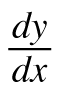. Homework Help ✎

1. y = sin−1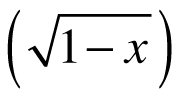2.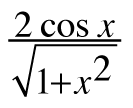3. y = xesec x

4. ln y = x ln x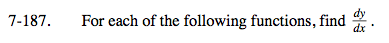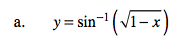Be mindful about positive and negative values.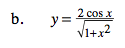Quotient Rule.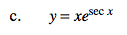Chain Rule.

$\text{Recall that }\frac{d}{dx}\text{ sec}x=\text{sec}x\text{tan}x$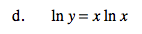Implicit differentiation.